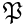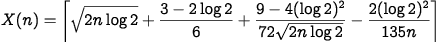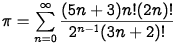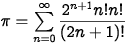﻿ Manuscripts
 Manuscripts David BrinkYou are welcome to contact me if you are interested in offprints of the published papers. 1. Der Fächer der Lady Windermere (German translation of Oscar Wilde’s Lady Windermere’s Fan), Projekt Gutenberg, 2003. 2. On alternating and symmetric groups as Galois groups, Israel Journal of Mathematics 142 (2004), 47–60. Fix an integer n ≥ 3. We show that the alternating group An appears as Galois group over any Hilbertian field of characteristic different from 2. In characteristic 2, we prove the same when n is odd. We show that any quadratic extension of Hilbertian fields of characteristic different from 2 can be embedded in an Sn-extension (i.e., a Galois extension with the symmetric group Sn as Galois group). For n ≥ 6, it will follow that An has the so-called GAR-property over any field of characteristic different from 2. Finally, we show that any polynomial f = Xn + … + a1X + a0 with coefficients in a Hilbertian field K whose characteristic doesn't divide n(n – 1) can be changed into an Sn-polynomial f* (i.e., the Galois group of f* over K, Gal(f*, K), is Sn) by a suitable replacement of the last two coefficients a0 and a1. These results are all shown using the Newton polygon. 3. On Zp-embeddability of cyclic p-class fields, Comptes Rendus Mathématiques de l'Académie des Sciences, La Société Royale du Canada 27 (2005), 48–53. It is investigated when a cyclic p-class field of an imaginary quadratic number field can be embedded in an infinite pro-cyclic p-extension. 4. Procyclic Galois Extensions of Number Fields, Ph.D. thesis, University of Copenhagen, 2006. 5. New light on Hensel’s lemma, Expositiones Mathematicae 24 (2006), 291−306. The historical development of Hensel's lemma is briefly discussed (section 1). Using Newton polygons, a simple proof of a general Hensel's lemma for separable polynomials over Henselian fields is given (section 3). For polynomials over algebraically closed, valued fields, best possible results on continuity of roots (section 4) and continuity of factors (section 6) are demonstrated. Using this and a general Krasner's lemma (section 7), we give a short proof of a general Hensel's lemma and show that it is, in a certain sense, best possible (section 8). All valuations here are non-Archimedean and of arbitrary rank. The article is practically self-contained. 6. Prime decomposition in the anti-cyclotomic extension, Mathematics of Computation 76 (2007), 2127−2138. For an imaginary quadratic number field K and an odd prime number l, the anti-cyclotomic Zl-extension of K is defined. For primesof K, decomposition laws forin the anti-cyclotomic extension are given. We show how these laws can be applied to determine if the Hilbert class field (or part of it) of K is Zl-embeddable. For some K and l, we find explicit polynomials whose roots generate the first step of the anti-cyclotomic extension and show how the prime decomposition laws give nice results on the splitting of these polynomials modulo p. The article contains many numerical examples. 7. Simultaneous diagonal equations over-adic fields, Acta Arithmetica 132 (2008), 393−399. Joint work with H. Godinho and P. Rodrigues. Consider a system of R diagonal forms of degree k in N variables over a-adic field K. Write k = pτm where p is the residue field characteristic and (m, p) = 1. We prove that this system has a non-trivial-adic solution if N exceeds (Rk)2τ+5, a bound independent of the field K. We prove the same if N exceeds 4nR2k2 where n = [K : Qp]. Both results improve previously known bounds. 8. Essentials of Statistics (103 pages), Bookboon, 2008. 9. Essentials of Statistics: Exercises (58 pages), Bookboon, 2008. 10. Five peculiar theorems on simultaneous representation of primes by quadratic forms, Journal of Number Theory 129 (2009), 464−468. It is a theorem of Kaplansky that a prime p ≡ 1 (mod 16) is representable by both or none of x2 + 32y2 and x2 + 64y2, whereas a prime p ≡ 9 (mod 16) is representable by exactly one of these binary quadratic forms. In this paper five similar theorems are proved, and a heuristic argument is given why there are no other results of the same kind. The latter argument relies on the (plausible) conjecture that the list of 485 known negative discriminants Δ such that the class group C(Δ) has exponent 4 is complete. The methods are purely classical. Video abstract. 11. Two theorems of Glaisher and Kaplansky, Functiones et Approximatio Commentarii Mathematici 41 (2009), 163–165. It is well known that, for any n > 0, the prime numbers of the form x2 + ny2 can be described by congruence conditions if and only if n is one of Euler's convenient numbers. Since 1, 2, 4, 8 and 16 are the only convenient powers of 2, the following theorem of Kaplansky is remarkable: A prime p ≡ 1 (mod 16) is representable by both or none of x2 + 32y2 and x2 + 64y2, whereas a prime p ≡ 9 (mod 16) is representable by exactly one of these forms. We give three new proofs of Kaplansky's theorem and use it to revive an old, seemingly forgotten result of Glaisher. 12. Remark on infinite unramified extensions of number fields with class number one, Journal of Number Theory 130 (2010), 304−306. We modify an idea of Maire to construct biquadratic number fields with small root discriminant, class number one, and having an infinite, necessarily non-solvable, strictly unramified Galois extension. It is indicated how to construct real quadratic number fields with the same properties. 13. Hölder continuity of roots of complex and p-adic polynomials, Communications in Algebra 38 (2010), 1658–1662. Let f be a polynomial with coefficients in an algebraically closed, valued field. We show a refinement of the principle of continuity of roots, namely, that each root α of f is locally Hölder continuous of order 1/μ as a function of the coefficients of f, where μ is the root multiplicity of α. This is derived as a consequence of a principle that could be called continuity of factors, namely, that if f = gh is a factorisation with (g, h) = 1, then the coefficients of g and h are locally Lipschitz continuous as functions of the coefficients of f. The proofs are elementary and of an algebraic nature. 14. Principal forms X2 + nY2 representing many integers, Abhandlungen aus dem Mathematischen Seminar der Universität Hamburg 81 (2011), 129–139. Joint work with P. Moree and R. Osburn. In 1966, Shanks and Schmid investigated the asymptotic behavior of the number B(x) of positive integers less than or equal to x which are represented by the quadratic form X2 + nY2, and showed that B(x) ~ C(n)∙x∙(log x)–1/2 with a constant C(n). Based on some numerical computations, they observed that the largest C(n) appears to be C(2) = 0.8728. In this paper, we prove that in fact the constant C(n) is unbounded (although in a very slow manner) as n runs through positive integers with a fixed number of prime divisors. Also, we find an explicit n with C(n) > C(2), namely, n = 13779962790518414129 for which C(n) = 0.8759. 15. The inverse football pool problem, Journal of Integer Sequences 14 (2011), 1–9. The minimal number of spheres (without "interior") of radius n required to cover the finite set {0,…, q – 1}n equipped with the Hamming distance is denoted by T(n, q). The only hitherto known values of T(n, q) are T(n, 3) for n = 1,…, 6. These were all given in the 1950s in the Finnish football pool magazine Veikkaaja along with upper and lower bounds on T(n, 3) for n ≥ 7. Recently, Östergård and Riihonen found improved upper bounds on T(n, 3) for n = 9, 10, 11, 13 using tabu search. In the present paper, a new method to determine T(n, q) is presented. This method is used to find the next two values of T(n, 3) as well as six non-trivial values of T(n, q) with q > 3. It is also shown that, modulo equivalence, there is only one minimal covering of {0, 1, 2}n for each n ≤ 7, thereby proving a conjecture of Östergård and Riihonen. For reasons discussed in the paper, it is proposed to denote the problem of determining the values of T(n, 3) as the inverse football pool problem. 16. Rédei fields and dyadic extensions, International Journal of Number Theory 7 (2011), 231–240. For an arbitrary non-square discriminant D, the Rédei field Γ0(D) is introduced as an extension of K = Q(√D) analogous to the genus field and connected with the Rédei-Reichardt Theorem. It is shown how to compute Rédei fields, and this is used to find socles of dyadic extensions of K for negative D. Finally, a theorem and two conjectures are presented relating the fields Q(√(–p)) and Q(√(–2p)) for an odd prime p. 17. Chevalley's theorem with restricted variables, Combinatorica 31 (2011), 127–130. First, a generalization of Chevalley's classical theorem from 1936 on polynomial equations f(x1,…, xN) = 0 over a finite field K is given, where the variables xi are restricted to arbitrary subsets Ai ⊆ K. The proof uses Alon's Nullstellensatz. Next, a theorem on integer polynomial congruences f(x1,…, xN) ≡ 0 (mod pν) with restricted variables is proved, which generalizes a more recent result of Schanuel. Finally, an extension of Olson's theorem on zero-sum sequences in finite Abelian p-groups is derived as a corollary. 18. Incan and Mayan Mathematics. Article in Encyclopedia of Mathematics and Society, Salem Press, Pasadena, CA, 2011, pp. 493–496. 19. Numbers, Complex. Ibid., pp. 721–724. 20. Relativity. Ibid., pp. 853–855. 21. A (probably) exact solution to the Birthday Problem, The Ramanujan Journal 28 (2012), 223–238. Given a year with n ≥ 1 days, the Birthday Problem asks for the minimal number X(n) such that in a class of X(n) students, the probability of finding two students with the same birthday is at least 50 percent. We derive heuristically the exact formulaand argue that the probability that a counter-example to this formula exists is less than one in 45 billion. We then give a new derivation of the asymptotic expansion of Ramanujan's Q-function and note its curious resemblance to the formula for X(n). 22. The spiral of Theodorus and sums of zeta-values at the half-integers, The American Mathematical Monthly 119 (2012), 779–786. The total angular distance traversed by the spiral of Theodorus is governed by the Schneckenkonstante K introduced by Hlawka. The only published estimate of K is the bound K ≤ 0.75. We express K as a sum of Riemann zeta-values at the half-integers:and compute it to 100 decimal places. We find similar formulas involving the Hurwitz zeta-function for the analytic Theodorus spiral and the Theodorus constant introduced by Davis. 23. A solution to the Basel Problem that uses Euclid's Inscribed Angle Theorem, Mathematics Magazine 87 (2014), 222–224. We present a short, rigorous solution to the Basel Problem that uses Euclid's Inscribed Angle Theorem (Proposition 20 in Book III of the Elements) and can be seen as an elaboration of an idea of Leibniz communicated to Johann Bernoulli in 1696. 24. Nilakantha's accelerated series for π (2014). To appear in Acta Arithmetica. We show how the idea behind a formula for π discovered by the Indian mathematician and astronomer Nilakantha (1445–1545) can be developed into a general series acceleration technique which, when applied to the Gregory-Leibniz series, gives the formulawith convergence as 13.5–n, in much the same way as the Euler transformation giveswith convergence as 2–n. Similar transformations lead to other accelerated series for π, including three "BBP-like" formulas, all of which are collected in an appendix. Optimal convergence is achieved using Chebyshev polynomials. 25. A proof without words of the Law of Cosines (2014). To appear in Eureka.